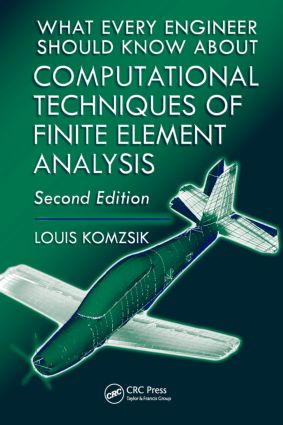# What Every Engineer Should Know about Computational Techniques of Finite Element Analysis

## 2nd Edition

CRC Press

350 pages | 68 B/W Illus.

##### Purchasing Options:\$ = USD
Hardback: 9781439802946
pub: 2009-04-28
SAVE ~\$24.00
\$120.00
\$96.00
x
eBook (VitalSource) : 9780429130274
pub: 2016-04-19
from \$60.00

FREE Standard Shipping!

### Description

Finite element analysis (FEA) has become the dominant tool of analysis in many industrial fields of engineering, particularly in mechanical and aerospace engineering. This process requires significant computational work divided into several distinct phases. What Every Engineer Should Know About Computational Techniques of Finite Element Analysis offers a concise, self-contained treatment of FEA and all of the tools needed for efficient use and practical implementation.

This book provides you with a walk-through of the process from the physical model to the computed solution. Based on the author's thirty years of practical experience in finite element analysis in the shipbuilding, aerospace, and automobile industries, it describes the transformation of the physical problem into a mathematical model, reduction of the model to a more efficient, numerically solvable form, and the solution of the problem using specific computational techniques. The author discusses time and frequency domain solutions as used in practice, as well as the representation of the computed results.

What Every Engineer Should Know About Computational Techniques of Finite Element Analysis serves as a to-the-point guide to using or implementing FEA for both beginners and everyday users who must apply the finite element method to your daily work. The techniques can be easily executed in most available FEA software packages.

CRC Press Authors Speak

Louis Komzsik introduces you to two books that share a common mathematical foundation, the finite element analysis technique. Watch the video.

### Reviews

"It is an excellent presentation really of what engineers should know about computational techniques in FEA. The descriptions of the various subjects are very clear and transparently expose the facts.

"I hope engineers are interested to learn these lectures, which give them the opportunity to take a critical position in their doing.

"This is necessary because

- computing uses floating point models while classical theories use inductive and deductive models

- computing is essentially finite while mathematics is the science of infinite

- computing sometimes promotes logical mistakes

"Also in view of these more or less philosophical aspects your book will sharpen the thinking about limitations of application of the FE technique."

-Dr. Otto Gartmeier, Manager, NVH Optimization, Daimler Chrysler Corporation

" I wish this book had been published earlier! …If you use NASTRAN on a daily basis as the Number 1 Code, you will find that Dr. Komzsik's book is unique and outstanding, compared to all other Finite Element books. Look at the real life examples. It shows that Dr. Komzsik studied mathematics and then was, for over 20 years, one of the team leaders at MSC developing and maintaining NASTRAN. All of the important features in real life applications are explained in a few sentences and illustrated if necessary…

"I highly recommend this excellent book for every engineer. Even for students, it is very affordable and should be used as a standard reference whenever a Finite Element code is applied."

-Dr. Ortwin Ohtmer, Professor of Mechanical Engineering, California State University, Long Beach, USA

NUMERICAL MODEL GENERATION

Finite Element Analysis

Solution of Boundary Value Problems

Finite Element Shape Functions

Finite Element Basis Functions

Assembly of Finite Element Matrices

Element Matrix Generation

Local to Global Coordinate Transformation

A Quadrilateral Finite Element

References

Finite Element Model Generation

Spline Approximation

Geometric Modeling Objects

Geometric Model Discretization

Delaunay Mesh Generation

References

Modeling of Physical Phenomena

Lagrange's Equations of Motion

Continuum Mechanical Systems

Finite Element Analysis of Elastic Continuum

A Tetrahedral Finite Element

Equation of Motion of Mechanical System

Transformation to Frequency Domain

References

Constraints and Boundary Conditions

The Concept of Multi-Point Constraints

The Elimination of Multi-Point Constraints

The Axial Bar Element

The Concept of Single Point Constraints

The Elimination of Single Point Constraints

References

Singularity Detection of Finite Element Models

Local Singularities

Global Singularities

Massless Degrees of Freedom

Industrial Case Studies

References

COMPUTATIONAL REDUCTION TECHNIQUES

Matrix Factorization and Linear System Solution

Finite Element Matrix Reordering

Sparse Matrix Factorization

Multifrontal Factorization

Linear System Solution

Distributed Factorization and Solution

Factorization Case Study

References

Static Condensation

Single Level, Single Component Condensation

Computational Example

Single Level, Multiple Component Condensation

Multiple Level Static Condensation

Static Condensation Case Study

References

Spectral Computations

Spectral Transformation

Lanczos Reduction

Generalized Eigenvalue Problem

Eigenvalue Computation

Distributed Eigenvalue Computation

Normal Modes Analysis Case Study

Complex Spectral Computations

Complex Modes Analysis Case Study

Dense Eigenvalue Analysis

Householder Reduction Techniques

Tridiagonal Reduction

Reduction to Hessenberg Form

References

Dynamic Reduction

Single Level, Single Component Dynamic Reduction

Accuracy of Dynamic Reduction

Computational Example

Single Level, Multiple Component Dynamic Reduction

Multiple Level Dynamic Reduction

Multibody Analysis Application

References

Component Modal Synthesis

Single Level, Single Component Modal Synthesis

Mixed Boundary Component Mode Reduction

Computational Example

Single Level, Multiple Component Modal Synthesis

Multiple Level Modal Synthesis

Component Modal Synthesis Case Study

References

ENGINEERING SOLUTION COMPUTATIONS

Modal Solution Technique

Modal Reduction

Truncation Error in Modal Reduction

The Method of Residual Flexibility

The Method of Mode Acceleration

Coupled Modal Solution Application

References

Transient Response Analysis

The Central Difference Method

The Newmark Method

Starting Conditions and Time Step Changes

Stability of Time Integration Techniques

Transient Solution Case Study

References

Frequency Domain Analysis

Direct Frequency Response Analysis

Reduced Order Frequency Response Analysis

Accuracy of Reduced Order Solution

Frequency Response Case Study

References

Nonlinear Analysis

Introduction to Nonlinear Analysis

Newton-Raphson Methods

Quasi-Newton Iteration Techniques

Convergence Criteria

Computational Example

Nonlinear Dynamics

References

Sensitivity and Optimization

Design Sensitivity

Design Optimization

Planar Bending of the Bar

Computational Example

Eigenfunction Sensitivities

Variational Analysis

References

Engineering Result Computations

Displacement Recovery

Stress Calculation

Nodal Data Interpolation

Level Curve Computation

Engineering Results Case Study

References

Closing Remarks

Annotation

Index

### About the Author

#### Louis Komzsik

Huntington Beach, CA, USA